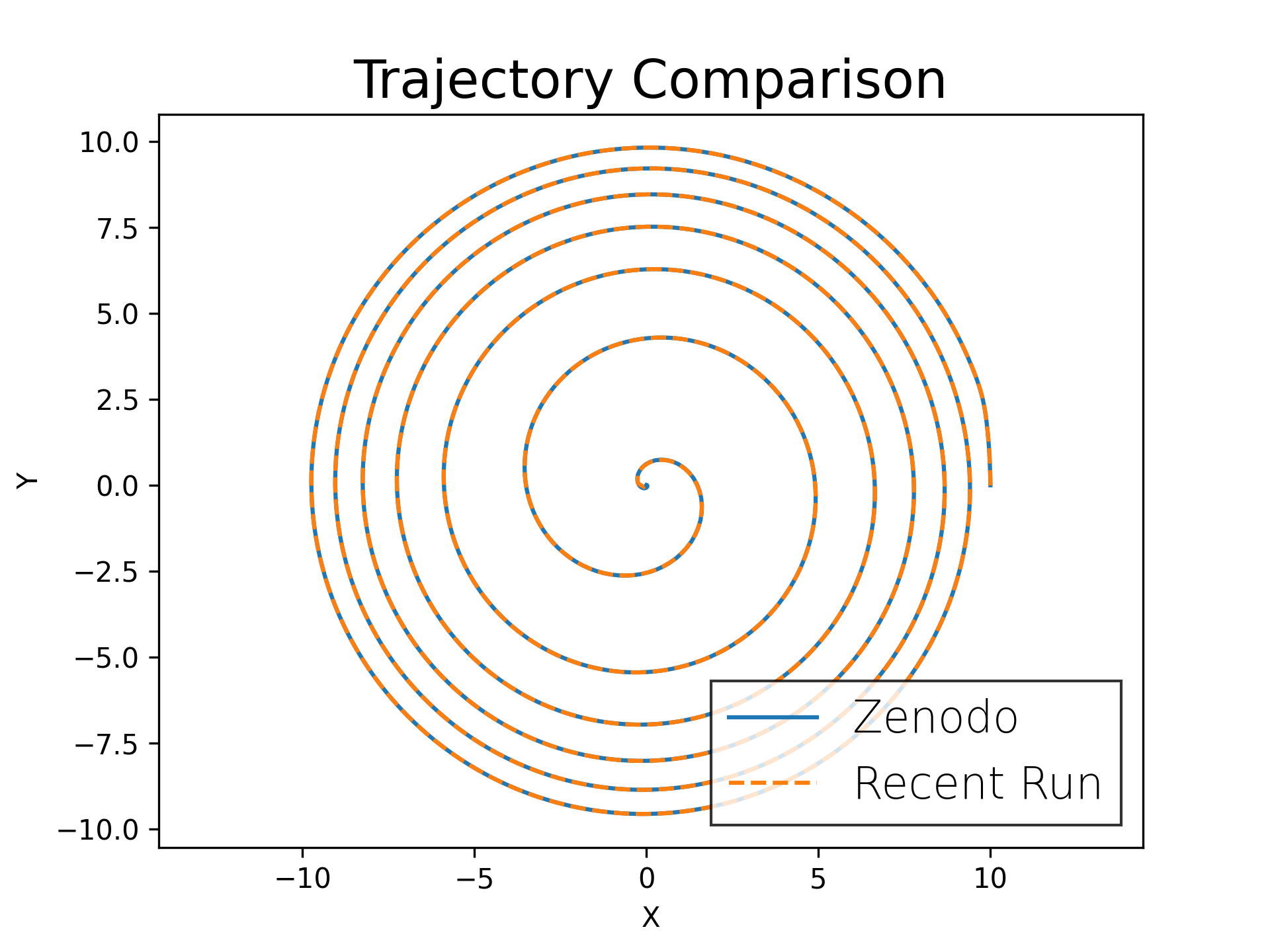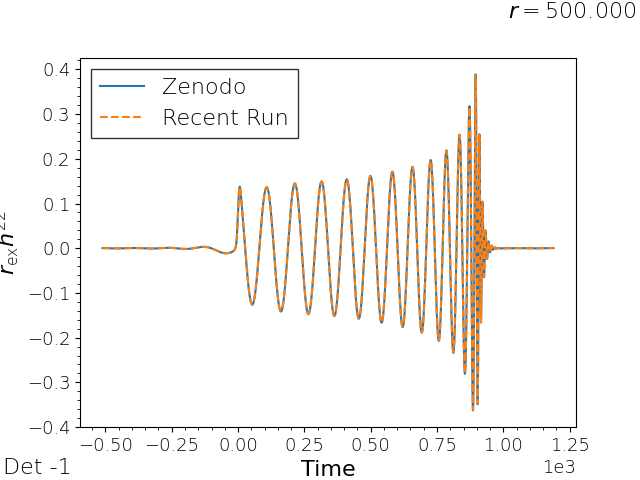# Generate plots for the binary black hole GW150914 simulation

## Using Mathematica

### Horizon coordinate trajectories

To plot the trajectory using Mathematica, download the Trajectories code and simply run

```        wolframscript -script trajectories.m --simulations-directory ~/simulations
```

In addition, this code also outputs the trajectory data as a simple csv file. You can then compare the output from the simulation with the reference trajectory using this python script:

### Gravitational waveform

The gravitational wave strain plot can be similarly produced using this Mathematica script:

```        wolframscript -script waveform.m --simulations-directory ~/simulations
```

To compare the output waveform data, use this python script and this csv file for the reference data.

## Using Kuibit

Alternatively, the comparison plots can be created with Kuibit. Kuibit can be installed as follows:

```        python3 -m pip install -U --user pip
python3 -m pip install -U git+https://github.com/Sbozzolo/kuibit.git
python3 -m pip install -U tikzplotlib
python3 -m pip install -U requests
```

Once the above are installed, the trajectories.py and waveform.py scripts can be run as follows:

```        python3 trajectories.py --datadir /work/USER/simulations/GW150914_28/